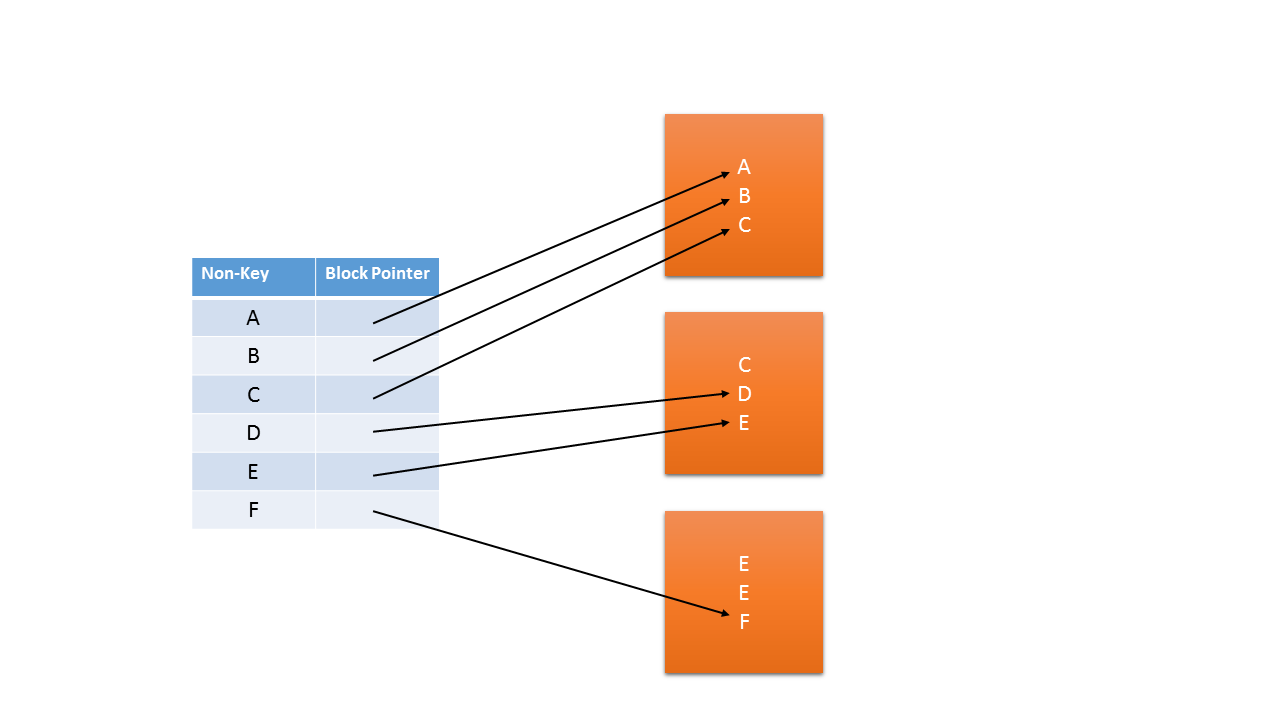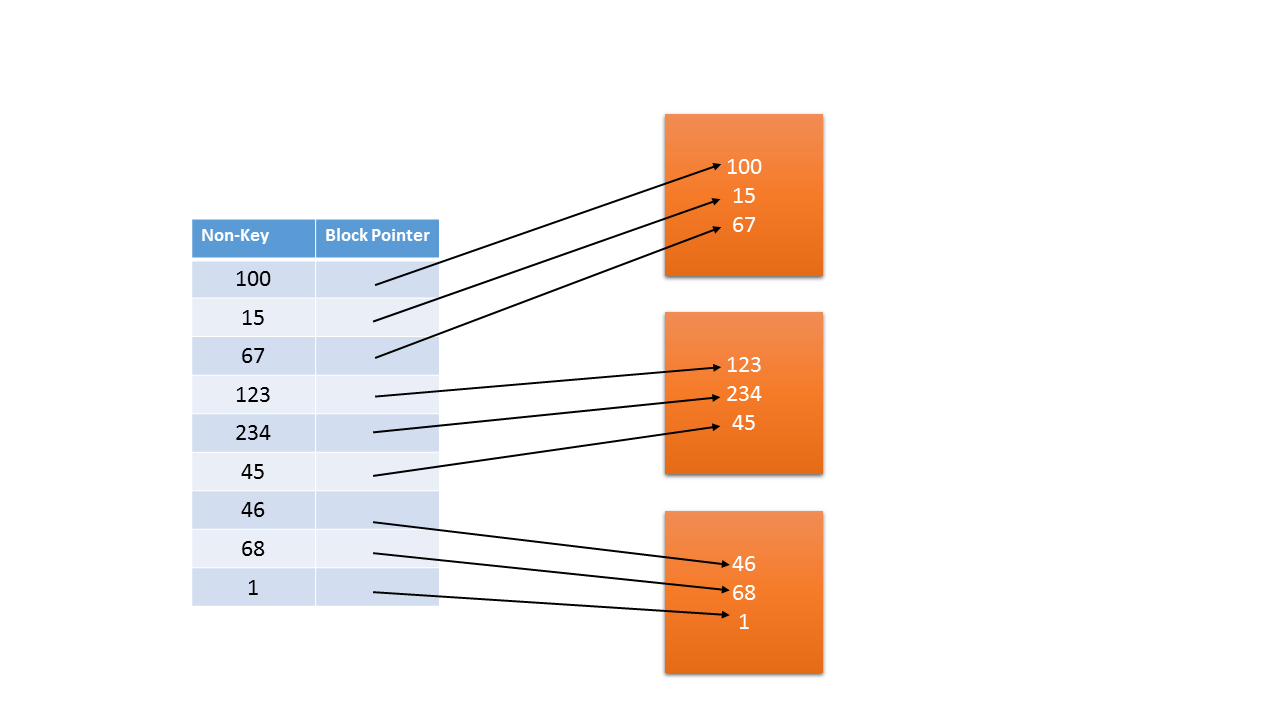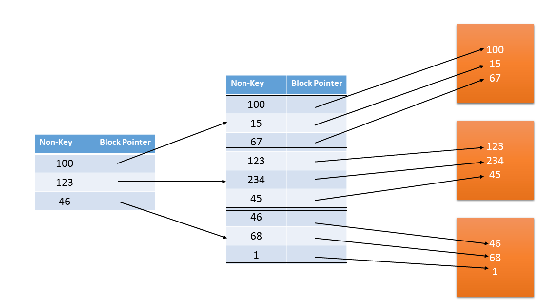# Classification of Indexing

In the previous page we have seen one classification. Here we are going to discuss one more classification.

• Dense Index: For every records we are having one entry in the index file.
• Sparse Index: Only one record for each block we will have it in the index file.

Primary Index (Primary Key + Ordered Data) A primary index is an ordered file whose records are fixed length size with 2 fields. First field is same as primary key and the second field is pointer to the data block.• Here index entry is created for first record of each block, called ‘block anchor’.
• Number of index entries thus are equal to number of blocks
• Average number of block accesses are = logB (worst case) + 1 (best case),
So on average it will be O(logB)
• The type of index is called sparse index because index is not created for all the records, only the first records of every block the entry is made into the index file.

Example on Primary Index

Number of Records = 30000, Block Size = 1024 Bytes, Strategy = Unspanned, Record Size = 100 Bytes,
Key Size = 6 Bytes, Pointer Size = 9 Bytes, Then find average number of block accesses with or without
index.

Data Records that can fit in 1 Block = 1024/100 = 10.24 block but we have to take the whole number so
we have to take only 10 records.
10 records can put in 1 block,
Total records = 30000, so total number of blocks = 30000/10 = 300 Blocks.
So without index we need ceil [log3000] = 12 blocks to access for finding the record. With Index Size of index = 15 Bytes (6+4)
Index Record / Block = floor [1024/15] = 68 [because strategy is unspanned]
Index Records = Number of data blocks = 3000
So no of index blocks = ceil (3000/68) = 45
So average number of block accesses = ceil [log45] + 1 = 6 + 1 = 7

### Clustered Indexing

Clustered Index is an ordered file with two fields, non-key and block pointer.
Clustering Index is created on data file whose file records are physically ordered on a non-key field which does not have distinct value for each record, that field is called clustering field.Explanation
• Index Entry is created for each distinct value of clustering field.
• Block pointer points to first block where key is available
• Type of index is dense and sparse both.

Average Number of block accesses required >= logB + 1

### Secondary Index

This a type of dense Index.

• Secondary Index provides a secondary means of accessing a file for which some primary access already exists.
• Secondary Index may be a key or a non-key
• Index entry is created for each record in data file
• Type of secondary index is Dense
Index fields are key (unordered) and block pointerExample on Secondary Index

Number of Records = 30000, Block Size = 1024 Bytes, Strategy = Unspanned, Record Size = 100 Bytes,
Key Size = 6 Bytes, Pointer Size = 9 Bytes, Then find average number of block accesses with or without
index.

Without Index
To search we need (30000 + 1) / 2 = 15001 Block accesses. With Index Size of index = 15 Bytes (6+4)
Index Record / Block = floor [1024/15] = 68 [because strategy is unspanned]
Index Records = Total Number of Records = 30000 So no of index blocks = ceil (30000/68) = 442
So average number of block accesses = ceil [log442] + 1 =

Example 2

#### Multi Level Indexing

Data Records = 16384, Size of Record = 32 Bytes, Storage Strategy = Unspanned, Block Size = 1024 Bytes, Size of key = 6 Bytes, Block Pointer = 10 Bytes

Pictorial Representation